Suche

# CO2MUCH - Solving the Problem

## Overview

Secondary School

Science, Physics, Chemistry

Sustainability

English

## Overview

Keywords: CO2, combustion, balanced equation, hydrogen
Disciplines: Physics, chemistry, science and technology
Age level of the students: 13-16
Time frame: 2-5 lessons
Partners: You can work together with the parallel class in your school or reach out to your local authorities.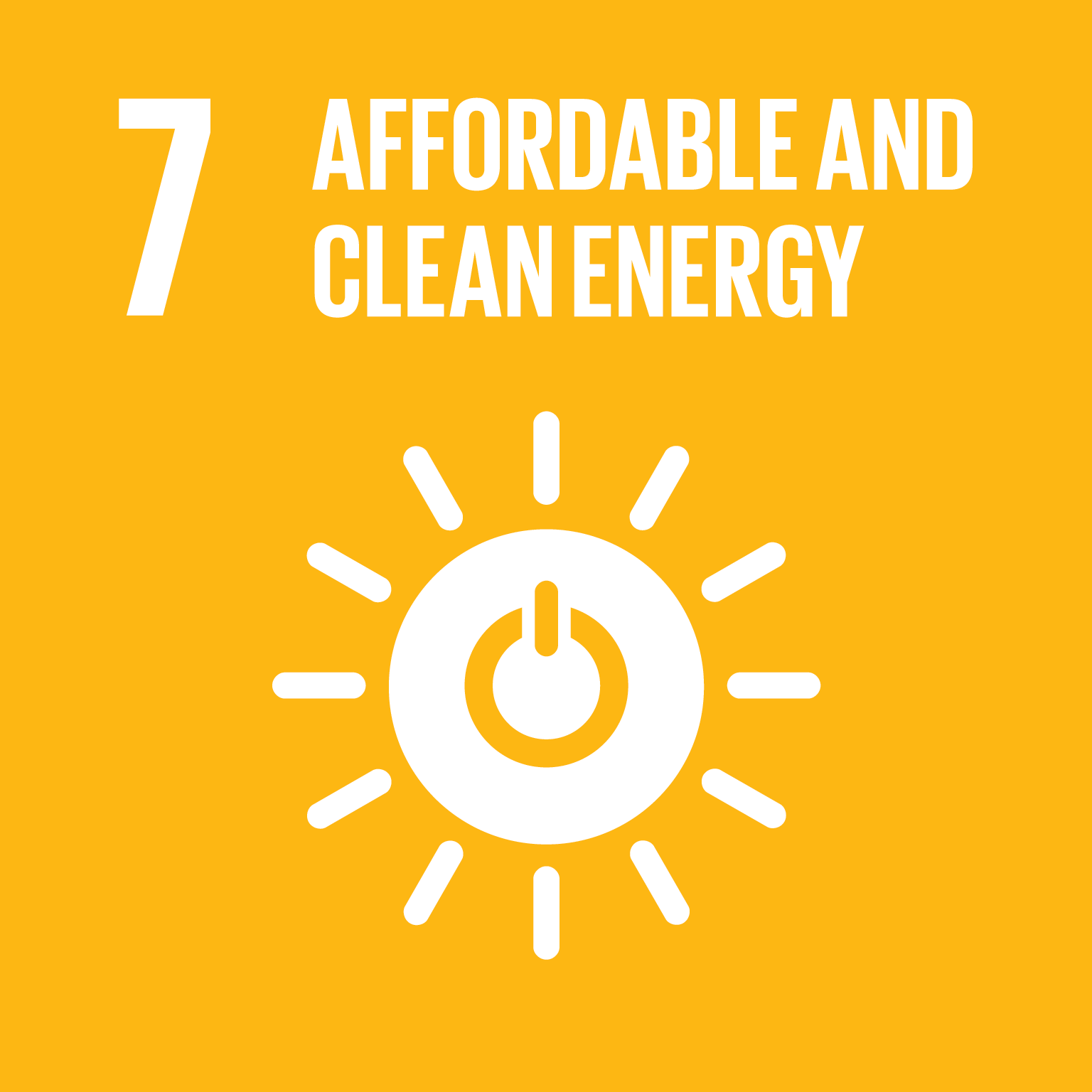### Summary

Hydrogen fuel cells have the advantage of not producing carbon dioxide. They produce electricity, heat and water from pure hydrogen and oxygen. Oxygen is abundant in the atmosphere, while hydrogen is the most common element in the universe. However, hydrogen does not exist on earth in its natural state. It therefore has to be produced artificially before it can be used as a fuel.

Students will look at the processes of producing hydrogen and how a fuel cell works. They study water as a source material for hydrogen fuel cells as an electrolyser to produce hydrogen with solar cells. Then they use the hydrogen fuel cell to produce electricity. They determine the energy efficiency by calculating the energy delivered to the cell during the electrolysis and the energy delivered by the cell while the motor is running.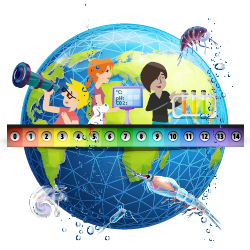### Hydrogen, a fuel for the future?

Students study three texts and diagrams and answer questions on hydrogen, how it is produced and the advantages and disadvantages of this fuel for the future and for solving the carbon dioxide problem.

CO2 emissions from the global transport sector reached nearly 8.5 Gt in 2019. The "zero net emissions by 2050" scenario requires that transport sector emissions decline by 20% to 5.7 Gt in 2030. This decline will depend on policies to encourage modal shifts to less carbon-intensive travel options, as well as operational and technical energy efficiency measures to reduce the carbon intensity of all modes of transport. Policies that promote the blending of true low-carbon fuels are critical to decarbonizing aviation, shipping, and heavy road freight.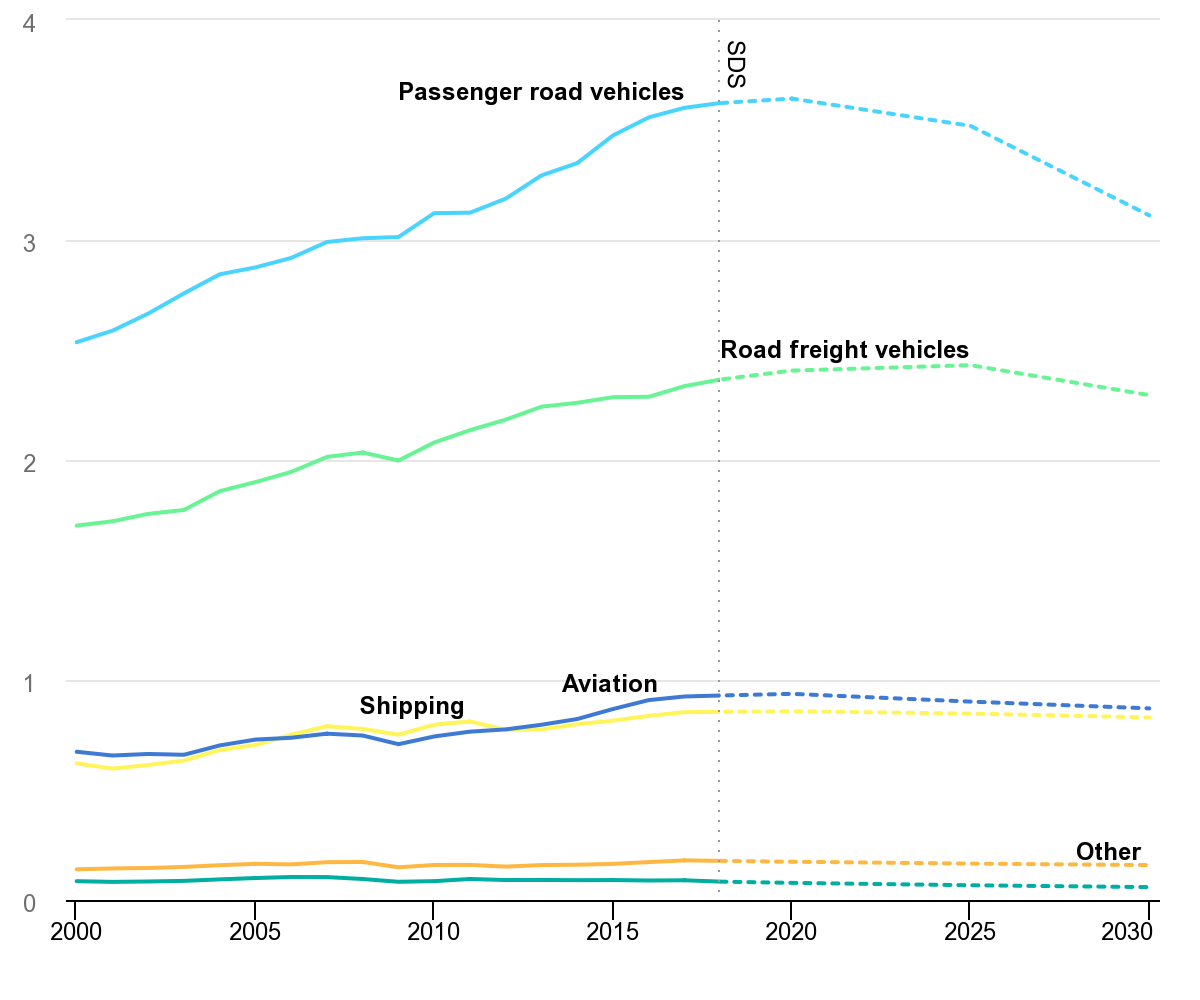IEA, Transport sector CO2 emissions by mode in the Sustainable Development Scenario, 2000-2030, IEA, Paris https://www.iea.org/data-and-statistics/charts/transport-sector-co2-emissions-by-mode-in-the-sustainable-development-scenario-2000-2030

1. What type of transportation produces CO2?
2. Estimate the mass of carbon dioxide emitted by passenger road vehicles in 2020.
3. Calculate the mass of carbon dioxide emitted by passenger road vehicles that is set to be produced in 2050.
4. Comment the «Zero net emission» expression.

It is very common to hear about hydrogen engines, hydrogen fuel cells and hydrogen cars. Hydrogen is used in the form of dihydrogen (H2).
A fuel cell stores the chemical energy of hydrogen combined with oxygen from the air. This chemical energy is converted and transferred into thermal energy and electrical energy used in hydrogen electric vehicles, where the hydrogen is combined with oxygen from the air.
In both cases the chemical transformation produces only water.

1. Identify the reactants and the product of the chemical transformation in hydrogen fuel cells.
2. Write the balanced equation of the transformation.
3. Complete the energy diagram with the correct energy form.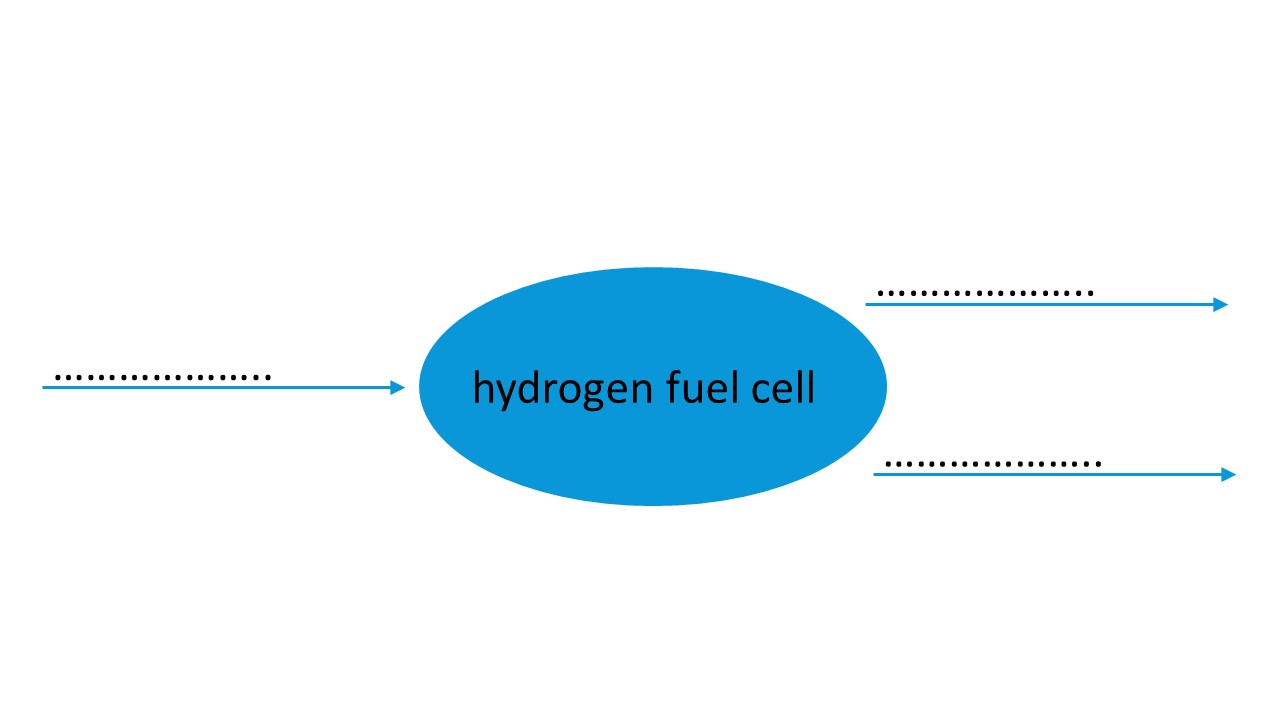Hydrogen does not exist in its natural state. It must be produced from hydrocarbons or water, which first requires an expenditure of energy. Hydrogen is mainly produced from fossil energy resources.

#### Fossil-based hydrogen - Creating hydrogen from hydrocarbons

The reforming of methane from natural gas is currently the main source of hydrogen. The reaction of methane with water at high temperature requires a lot of energy. It produces a mixture of carbon monoxide, carbon dioxide and hydrogen.

#### Green hydrogen - How can hydrogen by created using water electrolysis?

The passage of a direct current through water leads to its breakdown and the creation of hydrogen and oxygen: this is called water electrolysis. The breakdown of water is given by the following balanced equation:

2 H2O → 2 H2 + O2

1. Find the correctly balanced balance equation representing the methane reforming process and explain your choice.

• CH4 + H2O → CO2 + H2
• CH4 + H2O → CO2 + 3 H2
• CH4 + 2 H2O → CO2 + 4 H2 This one is the correct answer.

2. Balance the equation of water electrolysis.

H2O → H2 + O2

2. Under what conditions can fuel cells be considered "green" from an environmental point of view?

### How can hydrogen be produced using electrolysis?

Students learn about water electrolysis and the production of hydrogen. They discover the principal of electrolysis though a hydrogen fuel cell electrolyser using a solar cell. Hydrogen fuel cells can be bought in a set together with solar cells. Students measure voltage, amperage, volume of hydrogen produced and time. They can calculate energy consumption using the mathematical relationship E = IUt and write the chemical equation.

The students study the electrolysis of water through a hydrogen fuel electrolyser cell powered by a solar cell. You can download the protocol and tasks here as docx and pdf file.

#### Materials

• Solar fuel cell kit
• 400W lamp or sun
• Voltmeter
• Ammeter

#### Protocol

1. To assemble the fuel cell and the solar cell, follow the instructions that came with your solar fuel cell kit.
2. Measure the voltage, current, gas volume every 30 s.
3. Calculate the energy consumption.

#### At the anode (the positive pole)

Water reacts to form oxygen (O2) and hydrogen ions H+ and electrons e-. The hydrogen ions H+ pass through the proton exchange membrane (pem) to the cathode. The e- are blocked by the pem and travel through the electrical circuit.

#### At the cathode (the negative pole)

Hydrogen ions H+ and electrons e- coming from the electrical circuit recombine to form hydrogen (H2).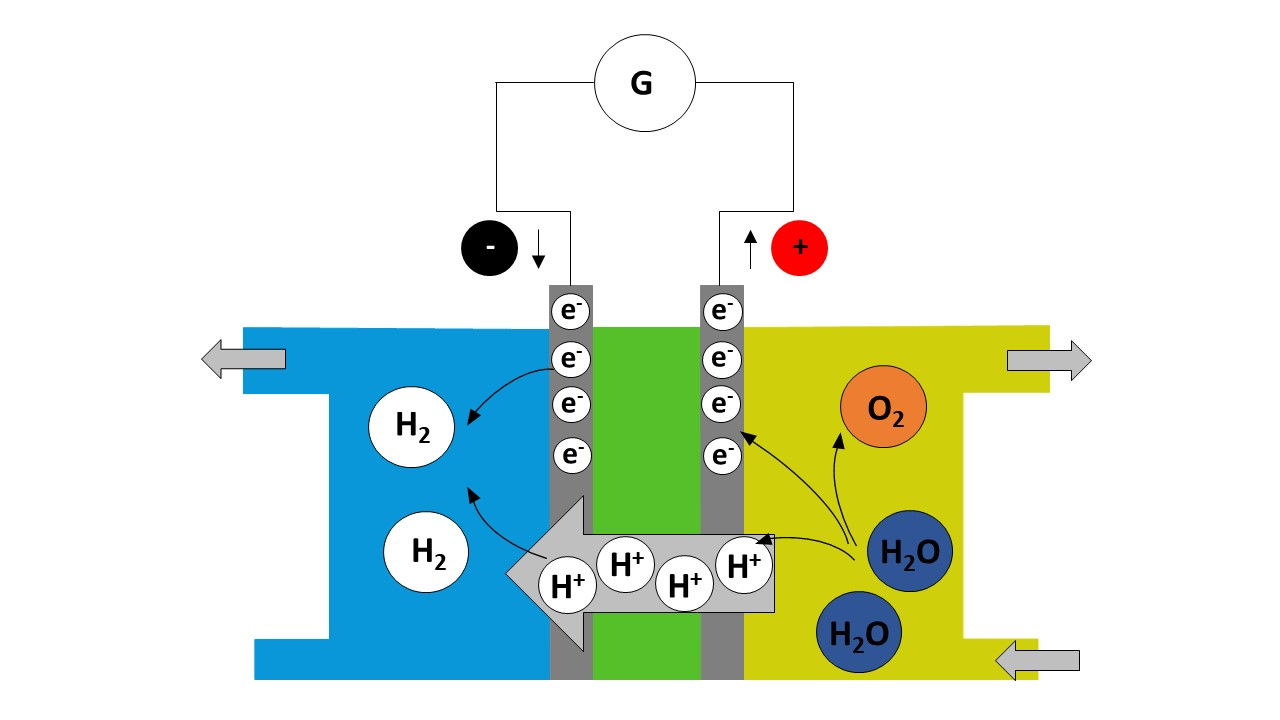The reaction observed is an electrochemical reaction, involving the following two redox couples:

H+(aq)/H2 (g) and O2(g)/H2O(l).

1. Write down the half-equation at the anode. Specify whether there is oxidation or reduction.
2. Write down the half-equation at the cathode. Specify whether there is oxidation or reduction.
3. Write the balance equation for the reaction used to operate the fuel cell.
4. Calculate the energy consumption.

### Hydrogen fuel cell, how does it work?

First the teacher shows fuel cells as generators and explains their working principle. Then students study the working principle of a hydrogen fuel cell. They can work on the five Hydrogen fuel cell H5P elements below or on a paper worksheet. You can download the worksheet here as docx and pdf file.

In the second part, the students also use a fuel cell kit to power an electrical motor.

#### At the anode (the negative pole)

Pressurised hydrogen gas (H2) with the platinum catalyst splits into hydrogen ions H+ and electrons e-.

The hydrogen ions H+ pass through the proton exchange membrane (pem) to the cathode.

The e- are blocked by the pem and travel through the electrical circuit to the cathode providing an electrical current.

#### At the cathode (the positive pole)

Oxygen (O2) (pure or coming from the air), hydrogen ions H+ and electrons e- (coming from the anode) recombine to form water molecules.

1. Label the fuel cell model to make the cell work.

The reaction observed is an electrochemical reaction, involving the following two redox couples:
H+(aq)/H2 (g) and O2(g)/H2O(l).

2. At the cathode, the positive pole of the cell, the oxidant involved is the oxygen of the pair O2 (g)/H2O (l).
Write down the half-equation at the cathode.
Specify whether there is oxidation or reduction.

• Redox half-equation: O2 (g) + 4 H+ (aq) + 4 e- = 2 H2O (l)
• At the cathode, there is a reduction of oxygen producing water.

3. At the anode, the negative pole of the cell, the reductant used is the hydrogen H2 of the pair H+ (aq) / H2 (g).
Write down the half-equation at the anode.
Specify whether there is oxidation or reduction.

• Redox half-equation: H2 (g) = 2 H+ (aq) + 2 e-
• At the anode, there is an oxidation of hydrogen.

4. Write the balanced equation for the reaction used to operate the fuel cell.

• The balanced equation for the reaction used to operate the fuel cell is as follows:
2 H2 (g) + O2 (g) = 2 H2O (l)

5. What material is recovered at the cathode?

• Water is recovered at the cathode.

After the students have studied the working principle of a fuel cell in theory, they can use a fuel cell kit to power an electrical motor. They measure voltage, current and hydrogen consumption in time and calculate energy consumption.

#### Materials

• One fuel cell per group
• Voltmeter
• Ammeter

1. Follow the instructions provided by the manufacturer to set up the fuel cell kit.
2. Connect the electrical motor, voltmeter and ammeter.
3. Measure voltage, current and gas consumption every 30 s.
4. Calculate the energy consumption.
5. Calculate the energy efficiency compared to the solar fuel cell electrolyser.

### Further ideas with hydrogen fuel cells

How about a car race? An interesting idea for another project is to build a car using the fuel cell for a hydrogen car race. You can set up a competition in class, against the parallel class or as a happening at your school.

### What can we do in our school?

To raise awareness, students can organise a car-free day with the help of the local authority. They can also propose to install solar panels or wind turbines to produce some electricity for their school.

1. International Energy Agency

2. For example from Horizon Educational but other producers are available.

Authors of CO2MUCH - Think Global, Act Local: Elena Poncela Blanco (ES), Philippe Mancini (FR)